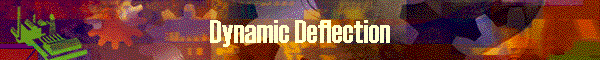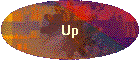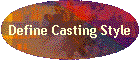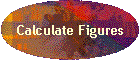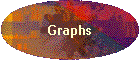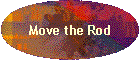### Calculating dynamics

DynaRod performs the calculation of rod moment value dynamically as its name implies.  For a question "Is there a dynamic type calculation for a static rod model, where the rod is not moving?", the answer is YES.  To understand this clearly, let's consider a rod which is fixed to the wall at its grip and which hangs a certain weight at the top of the rod.   The rod will start bending from the tip top and balance in a certain level with flexure.  DynaRod performs the calculation of its bend angles dynamically.  "To calculate dynamically" means that the moment value  "one second after" is calculated based on the shape of the rod "one second before".  This type of calculations are continued until the rod finally balances.

For a dynamic rod model, the dynamic calculation must assume that the rod is moving.   That is, when a rod moves in a certain time frame, for a certain distance and towards a certain direction, DynaRod calculates the receiving load for the rod, and calculates the deflection and stress as the result of movement,  dynamically from time to time.  At this time, it also calculate the values for "one moment after" situation based on the "one moment before" situation.

Then how does DynaRod assume and understand the rod movement?  Users of this tool can define or design the movement of a rod by using one of DynaRod features.  It is called as "definition of casting", or "design of casting style".  If a casting style is defined, rod movement can be automatically calculated for direction, time duration, and velocity, as a result, acceleration and so on.

In dynamic calculation, it divides a duration of time (mainly in the unit of second) into several continuous subdivided time blocks, called as "phase".  Within one block of time, calculation is made based on the deflected rod in the previous time block and this is repeated for all the subdivision of time.  So it spends a little computer time to complete the calculations for one casting style, then the resulted deflections and stress curves become very close to the   natural and actual deflections as if the rod is cast in actual fishing.

### Moving Rod

Calculation of moving rod is done as follows.

The picture below shows a rod at three points of time, which is moving from right to left with rotation.  For the ease of understanding, rod shape is straight.  It is because  we are now considering the cause of the deflection, not the result.

Among all the point of the rod, we just look at the top of the rod.  Tip top (I mean 1" section at the top of the rod), moves along with arrow-a during the time duration-1, then moves along with arrow-b in time duration-2.  Since, both of "a" and "b" are the movement during a certain time, we can have the concept of distance, velocity and direction by these arrows.  From those we can calculate Acceleration for both of movement "a" and "b".  As an acceleration is a vector which has direction and magnitude, we can have the equation of c = a + b.  As most of us knows, vectors can be composed and decomposed by the rule of a parallelogram, where the movement through "a" and "b" is equal to "c" in physics.

DynaRod can produce the movement of a rod by specifying the factors as follows;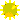Direction of movement, up/down/right/leftRotation of movement, clock wise/counter clock wiseDuration of time for movement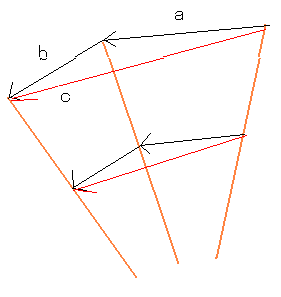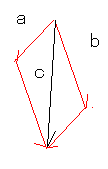DynaRod performs the calculation based on the specified movement for a rod for those matters in sequence;speed (velocity)accelerationforce of inertiamomentbend angledeflectionstress

### Direction of inertia

In dynamic calculation, DynaRod is distinguishing the directions of inertia for every part on a rod.  The picture below shows several examples of inertia and its directions.

The direction of inertia (acceleration) is different at each point of a rod even if the rod moves toward a certain direction and if rod angle is changes.  The picture at left show this.  The rod is rotated and moved downward.  In this case, tip top receives the force of inertia in direction of (a) but the end part of the rod in direction (b).

In the picture at right, the rod is simply rotated toward upward.  Let's assume that the rod tries to cast forward a fly line which is in the air and moving backward horizontally.  The fly line pulls the tip top toward right side and the direction is horizontal in this case.  But the top guide moves from down to up, then the top guide and the top section of the rod blank receives the force of inertia towards (top guide, bamboo blank) direction while Ferrule is receiving its own force of inertia.
DynaRod identifies all these forces of inertia and perform calculations.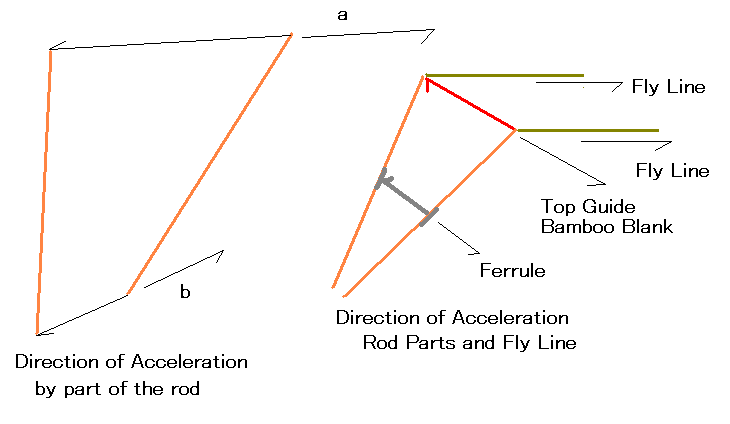To utilize this dynamic deflection function, user must decide how the rod should move on the [Define Casting Style] panel.  Other detailed matters are calculated by DynaRod.

Another important factor of realistic calculation is to take the Gravity Acceleration  into account.  DynaRod is adding the gravity acceleration in all the calculation of moment as well as the acceleration described above.
This is depicted that the horizontally held rod is bent by the weight (mass x gravity acceleration) of the rod components such as top guide, blanks, ferrules, and fly line.